For calculations, Lateral Surface Area means curved surface area. It's called lateral surface area for cube and cuboid and curved surface area for cylinder, cone and hemisphere.

The formulas for calculating Surface Area and Volume are

## Cube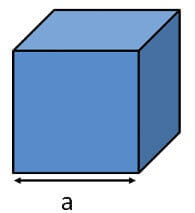• Lateral Surface Area = 4a 2
• Total Surface Area = 6a 2
• Volume = a 3

## Cuboid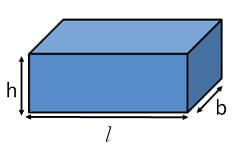• Lateral Surface Area = 2(lh + bh) = 2 (l + b) h
• Total Surface Area = 2(lb + bh + lh)
• Volume = l x b x h

## Cylinder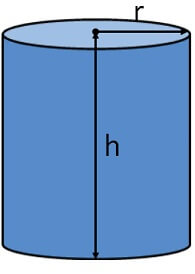• Curved Surface Area = 2πrh
• Total Surface Area = 2πrh + πr 2  + πr 2 = 2πrh + 2πr 2  = 2πr(r + h)
• Volume = πr 2 h

## Cone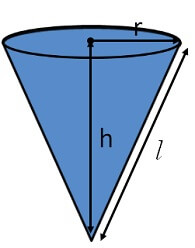• Curved Surface Area = πrl
• Total Surface Area = πrl + πr 2  = πr(r + l)
• Volume = 1/3 πr 2 h

## Hemisphere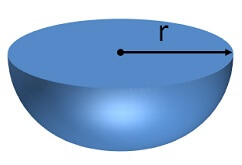• Curved Surface Area = 2πr 2
• Total Surface Area = 2πr 2 + πr 2  = 3πr 2
• Volume = 2/3 πr 3

## Sphere• Surface Area = 4πr 2
• Volume = 4/3 πr 3

## Frustum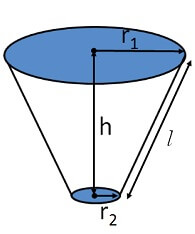• Curved Surface Area = π(r 1 + r 2 )l
• Total Surface Area =π(r 1 + r 2 )l + πr 1 + πr 2 2
• Volume = 1/3 πh (r 1 2 + r 2 2 + r 1 r 2 )
1. Chapter 13 Class 10 Surface Areas and Volumes (Term 2)
2. Serial order wise
3. Examples